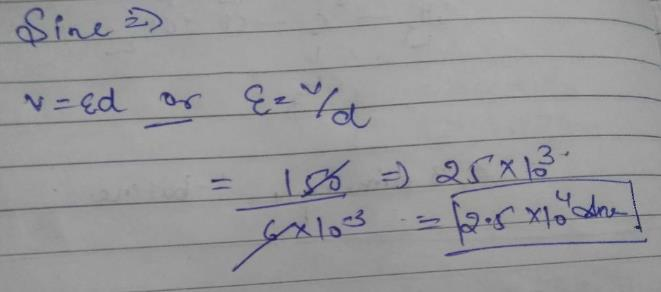Courses

# Test: Capacitors

## 10 Questions MCQ Test Physics Class 12 | Test: Capacitors

Description
This mock test of Test: Capacitors for JEE helps you for every JEE entrance exam. This contains 10 Multiple Choice Questions for JEE Test: Capacitors (mcq) to study with solutions a complete question bank. The solved questions answers in this Test: Capacitors quiz give you a good mix of easy questions and tough questions. JEE students definitely take this Test: Capacitors exercise for a better result in the exam. You can find other Test: Capacitors extra questions, long questions & short questions for JEE on EduRev as well by searching above.
QUESTION: 1

### Increasing the charge on the plates of a capacitor means

Solution:

Q = CV, where C is decided only by the geometrical factors

QUESTION: 2

### In a parallel plate capacitor the potential difference of 150 V is maintained between the plates. If distance between the plates is 6 mm, what will be the electric field at points A and B?​

Solution:QUESTION: 3

### Which of the following is a non polar dielectric?

Solution:

Alcohol and HCl are polar molecules since they have a net dipole moment towards a particular direction. Both water and benzene are non-polar molecules. But water is a conductor of electricity, whereas benzene is a dielectric (insulator).

QUESTION: 4

The distance between the plates of a capacitor is d. What will be the new capacitance if a metal plate of thickness d/2 is introduced between the plates without touching them

Solution:

Definition based

QUESTION: 5

What will be the effect on capacitance, if the distance between the parallel plates reduced to one-third of its original value?​

Solution:

C=(Kε0A/d)∝K/d
Hence,C1/C2=d2/d1=(d/3)d=3
C2=3C1

QUESTION: 6

What should be the radius of an isolated spherical conductor so that it has a capacity of 2μF?​

Solution:

Capacitance of an isolated spherical conductor =4π€R , where €=permittivity of vacuum
C=2×10-6F
C=4π€R
4×3.14×8.85×10-12×R=2×10-6
R=2×10-6/4×3.14×8.85×10-12
=1.8×104 m

QUESTION: 7

Work done in placing a charge of 8 x 10-18 C on a condenser of capacity 100 microfarad is​

Solution:

Given, charge =q=8×10−18C and Capacitance =C=100μF
Here the work done is the energy stored in the capacitor. i.e,
W=q2​/C= (8×10−18)2/100×10−6​=32×10−32J

QUESTION: 8

Capacitor preferred when there is a high frequency in the circuits is ______

Solution:

Mica capacitors are preferred for high frequency circuits because they have low ohmic losses and less reactance.

QUESTION: 9

A capacitor stores 0.24 coulombs at 10 volts. Its capacitance is

Solution:

Q = CV

QUESTION: 10

Work done in charging a capacitor is stored in it in the form of electrostatic energy, given by

Solution:

W = Energy stored in the capacitor

• Test